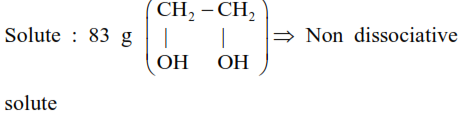# 83g of ethylene glycol dissolved

Question:

$83 \mathrm{~g}$ of ethylene glycol dissolved in $625 \mathrm{~g}$ of water. The freezing point of the solution is $\mathrm{K} .$ (Nearest integer)

[Use : Molal Freezing point depression constant of water $=1.86 \mathrm{~K} \mathrm{~kg} \mathrm{~mol}^{-1}$ ]

Freezing Point of water $=273 \mathrm{~K}$

Atomic masses : $\mathrm{C}: 12.0 \mathrm{u}, \mathrm{O}: 16.0 \mathrm{u}, \mathrm{H}: 1.0 \mathrm{u}]$

Solution:

$\mathrm{k}_{\mathrm{f}}=1.86 \mathrm{k} . \mathrm{kg} / \mathrm{mol}$

$\mathrm{T}_{\mathrm{f}}^{\mathrm{o}}=273 \mathrm{k}$

solvent : $\mathrm{H}_{2} \mathrm{O}(625 \mathrm{~g})$$\Rightarrow \Delta \mathrm{T}_{\mathrm{f}}=\mathrm{k}_{\mathrm{f}} \times \mathrm{m}$

$\Rightarrow\left(\mathrm{T}_{\mathrm{f}}^{\mathrm{o}}-\mathrm{T}_{\mathrm{f}}^{\mathrm{l}}\right)=1.86 \times \frac{83 / 62}{624 / 1000}$

$\Rightarrow 273-\mathrm{T}_{\mathrm{f}}^{1}=\frac{1.86 \times 83 \times 1000}{62 \times 625}=\frac{154380}{38750}$

$\Rightarrow 273-\mathrm{T}_{\mathrm{f}}^{1}=4$

$\Rightarrow \mathrm{T}_{\mathrm{f}}^{1}=259 \mathrm{~K}$